Computer Science‎ > ‎

### Python for Data: (9) Regularization & ridge regression with batch GD

Let's understand what the hell is regularization ?
When the model fits the training data but does not have a good predicting performance and generalization power, we have an over-fitting problem. Here is the best answer at quora.com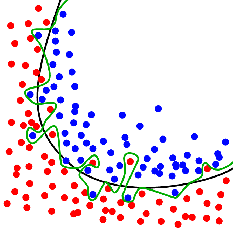Here one can see by using this visualization that the line separating red and blue dots (data points) have 2 classifier one is green line (over-fitting) another is black line (best-fit).

Regularization is a technique used in an attempt to solve the overfittingproblem in statistical models.*

First of all, I want to clarify how this problem of overfitting arises.

When someone wants to model a problem, let's say trying to predict the wage of someone based on his age, he will first try a linear regression model with age as an independent variable and wage as a dependent one. This model will mostly fail, since it is too simple.

Then, you might think: well, I also have the age, the sex and the education of each individual in my data set. I could add these as explaining variables.

Your model becomes more interesting and more complex. You measure its accuracy regarding a loss metric L(X,Y) where X is your design matrix and Y is the observations (also denoted targets) vector (here the wages).

You find out that your result are quite good but not as perfect as you wish.

So you add more variables: location, profession of parents, social background, number of children, weight, number of books, preferred color, best meal, last holidays destination and so on and so forth.

Your model will do good but it is probably overfitting, i.e. it will probably have poor prediction and generalization power: it sticks too much to the data and the model has probably learned the background noise while being fit. This isn't of course acceptable.

So how do you solve this?

It is here where the regularization technique comes in handy.

You penalize your loss function by adding a multiple of an L1 (LASSO) or an L2 (Ridge) norm of your weights vector w (it is the vector of the learned parameters in your linear regression). You get the following equation:

L(X,Y)+λN(w)

(L is either the L1L2 or any other norm)

This will help you avoid overfitting and will perform, at the same time, features selection for certain regularization norms (the  L1 in the LASSO does the job).

Finally you might ask: OK I have everything now. How can I tune in the regularization term  λ?

One possible answer is to use cross-validation: you divide your training data, you train your model for a fixed value of λ and test it on the remaining subsets and repeat this procedure while varying  λ. Then you select the best λ that minimizes your loss function.

Let's start and make our hands dirty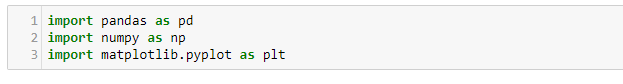This time we are working with ''wineQuality.csv'' data set available here.  Some useful information about data-set before proceeding further-

Attribute Information:

Input variables (based on physicochemical tests):
1 - fixed acidity
2 - volatile acidity
3 - citric acid
4 - residual sugar
5 - chlorides
6 - free sulfur dioxide
7 - total sulfur dioxide
8 - density
9 - pH
10 - sulphates
11 - alcohol
Output variable (based on sensory data):
12 - quality (score between 0 and 10)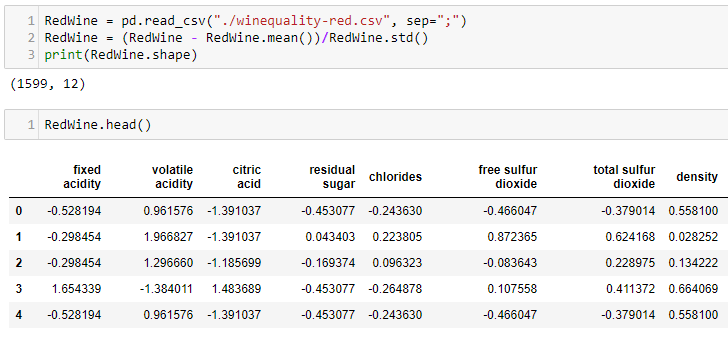Here we are loading the csv file into our python  notebook and normalizing it then printing it's shape. It has 12 columns and 1599 rows.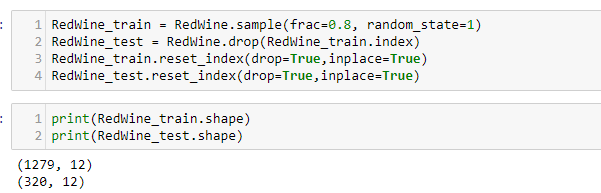As usual dividing data-set into train (80%) and test (20%).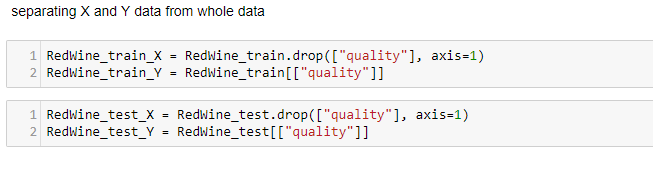Remember, almost everything in the world of Machine Learning will be processed in the form of Matrices.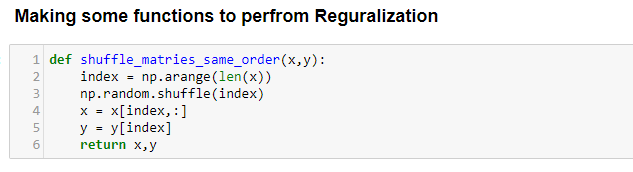Mini-batch gradient descent is a variation of the gradient descent algorithm that splits the training dataset into small batches that are used to calculate model error and update model coefficients.

Implementations may choose to sum the gradient over the mini-batch or take the average of the gradient which further reduces the variance of the gradient.

Mini-batch gradient descent seeks to find a balance between the robustness of stochastic gradient descent and the efficiency of batch gradient descent. It is the most common implementation of gradient descent used in the field of deep learning.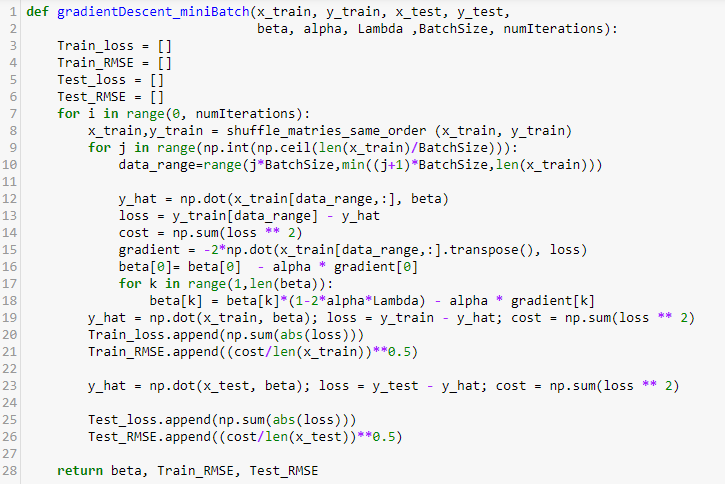This function is dedicated for Mini batch gradient descent. Remember we can so such operations with the help of scikit-learn in 2 lines of code. But that would not be a good idea for beginner.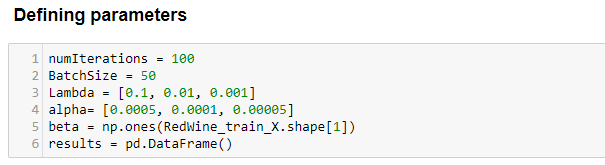Above we specified our parameters that every batch of gradient descent will adopt. Remember Lambda is the regularization parameter here. It has most influence on your results.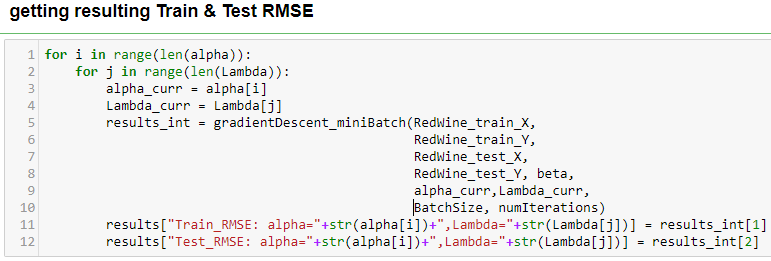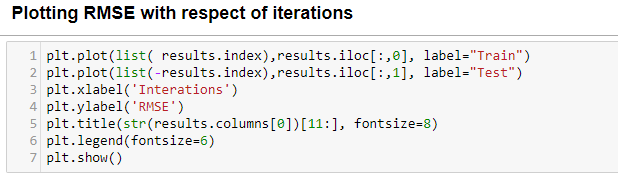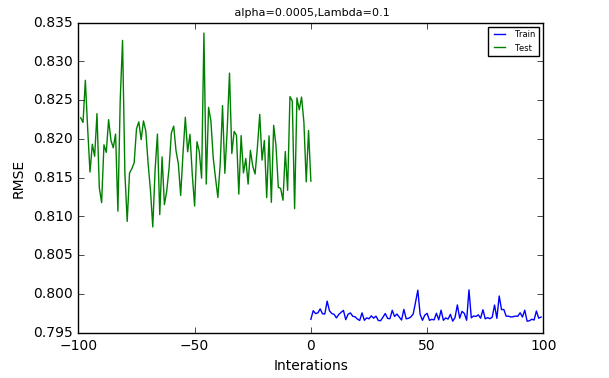See here  our alpha is 0.0005 and lambda = 0.1 we can see that there is over-fitting since our train and test both are jiggering. Let's try with different parameter values .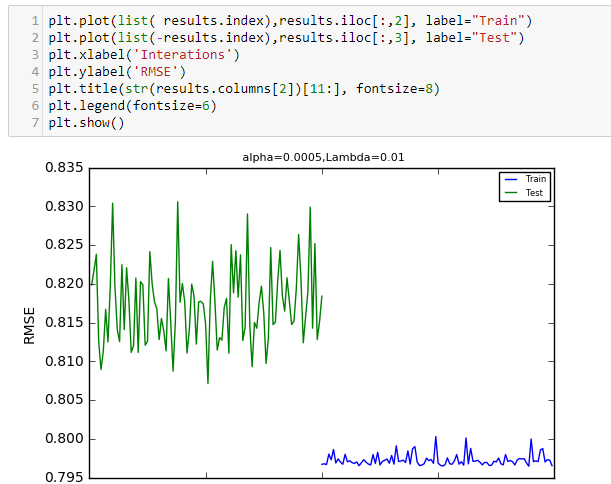Remember, here we are plotting in batches (see line 1 & 2 of code) by using 'iloc'

I have tried this with so many batches but let's hop up to the best parameter one.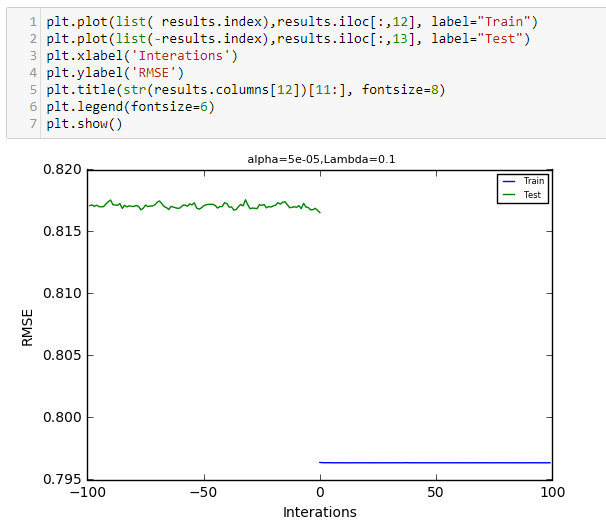Here our model is not over-fitted. It's the best fit on this data set. Lambda = 0.1 and alpha = 5e-05
So, machine learners here we learnt how to make our prediction accurate with-out letting model to learn the noise around data.

What's Next
• Hyper-parameter tuning and Cross validation